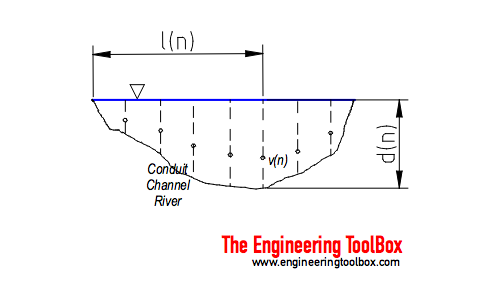Engineering ToolBox - Resources, Tools and Basic Information for Engineering and Design of Technical Applications!

# Velocity-Area Flowmetering

## Flow rate or discharge in an open conduit, channel or river can be calculated with the velocity-area principle.

The velocity-area principle is based on velocity measurements in a open flow like a conduit, channel or river.Velocities and depths across the stream are measured as indicated in the figure above. A partial discharge in a section of the stream can be calculated as

qn = vn an              (1)

where

qn = flow rate or discharge in section n    (m3/s, ft3/s)

vn = measured velocity in section n   (m/s, ft/s)

an = area of section n   (m2, ft2

One simple way to express the section area is

an = dn (ln+1 - ln-1) / 2                              (2)

The total flow in the stream can be summarized to

Q = Σ1n vn an                         (3)

where

Q = summarized flow rate or discharge in the conduit   (m3/s, ft3/s)

The accuracy of estimate depends on the profile of the conduit and the number of measurements. For conduits with regular shapes like rectangular channels a limited number of measurements are required. For irregular shapes - like natural rivers or similar - higher accuracy requires more measurements both horizontal and vertical.

### Example - Computing Flow Rate in a Channel

From a conduit we have three measurements:

Measured ValuesCalculated Values
nv
(m/s)
d
(m)
l
(m)
a
(m2)
q
(m3/s)
0 0 0 0
1 3 1 2 2 6
2 4 1.5 4 3 12
3 3 0.9 6 1.8 5.4
4 0 0 8
Summarized 23.4

The section areas can be calculated like

a1 = (1 m) ((4 m) - (0 m)) / 2

= 2 m2

a2 = (1.5 m) ((6 m) - (2 m)) / 2

= 3 m2

a3 = (0.9 m) ((8 m) - (4 m)) / 2

= 1.8 m2

The flow rates can be calculated as

q1 = (3 m/s) (2 m2)

= 6 m3/s

q2 = (4 m/s) (3 m2)

= 12 m3/s

q3 = (3 m/s) (1.8 m2)

= 5.4 m3/s

The total flow can be summarized as

Q = (6 m3/s) + (12 m3/s) + (5.4 m3/s)

= 23.4 m3/s

Note - there are alternative ways to calculate the section flow rates:

### Simple Average Method

Using the simple average of two successive vertical depths, their mean velocity, and the distance between them can be expressed as

qn to n+1  = [(vn + vn+1) / 2] [(dn + dn+1 ) / 2] (ln+1 - ln)                  (4)

### Midsection Method

With the midsection method, the depth and mean velocity are measured for each number of verticals along the cross section. The depth at a vertical is multiplied by the width, which extends halfway to the preceding vertical and halfway to the following vertical, to develop a cross-sectional area. The section flow rate can be expressed as

qn = vn [((ln - ln-1) + (ln+1 - ln)) / 2] dn                 (5)

## Related Topics

• Flow Measurement - Flow metering principles - Orifice, Venturi, Flow Nozzles, Pitot Tubes, Target, Variable Area, Positive Displacement, Turbine, Vortex, Electromagnetic, Ultrasonic Doppler, Ultrasonic Time-of-travel, Mass Coriolis, Mass Thermal, Weir V-notch, Flume Parshall and Sluice Gate flow meters and more.

## Engineering ToolBox - SketchUp Extension - Online 3D modeling!

Add standard and customized parametric components - like flange beams, lumbers, piping, stairs and more - to your Sketchup model with the Engineering ToolBox - SketchUp Extension - enabled for use with the amazing, fun and free SketchUp Make and SketchUp Pro .Add the Engineering ToolBox extension to your SketchUp from the SketchUp Pro Sketchup Extension Warehouse!

Translate

## Privacy

We don't collect information from our users. Only emails and answers are saved in our archive. Cookies are only used in the browser to improve user experience.

Some of our calculators and applications let you save application data to your local computer. These applications will - due to browser restrictions - send data between your browser and our server. We don't save this data.

## Citation

• Engineering ToolBox, (2017). Velocity-Area Flowmetering. [online] Available at: https://www.engineeringtoolbox.com/velocity-area-computing-flow-principle-d_1974.html [Accessed Day Mo. Year].

Modify access date.

. .

#### Scientific Online Calculator3 10